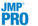Publication date: 06/16/2020

## Options for Ordinal Fits

The following options are available in the Ordinal Logistic Fit red triangle menu:

Save

Contains the following Save options:

Save Probability Formula

Creates columns in the current data table that contain formulas for linear combinations of the response levels, prediction formulas for the response levels, and a prediction formula giving the most likely response.

For an ordinal response model with r levels, JMP creates the following columns:

a column called Linear that contains the formula for a linear combination of the regressors without an intercept term

columns called Cum[j] that each contain a formula for the cumulative probability that the response is less than or equal to level j, for levels j = 1, 2, ... r - 1. There is no Cum[j = 1, 2, ... r - 1] that is 1 for all rows

columns called Prob[j = 1, 2, ... r - 1], for 1 < j < r that each contain the formula for the probability that the response is level j. Prob[j] is the difference between Cum[j] and Cum[j – 1]. Prob is Cum, and Prob[r] is 1 – Cum[r – 1].

a column called Most Likely response name that selects the most likely level of each row based on the computed probabilities.Publish Probability Formulas

Creates probability formulas and saves them as formula column scripts in the Formula Depot platform. If a Formula Depot report is not open, this option creates a Formula Depot report. See Formula Depot in Predictive and Specialized Modeling.

Save Quantiles

(Available only when the response is numeric and has the ordinal modeling type.) Creates columns in the current data table named OrdQ.05, OrdQ.50, and OrdQ.95 that fit the quantiles for these three probabilities.

Save Expected Value

(Available only when the response is numeric and has the ordinal modeling type.) Creates a column in the current data table called Ord Expected. This column contains the linear combination of the response values with the fitted response probabilities for each row and gives the expected value.